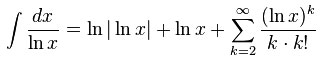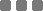## Wednesday, July 24, 2013

### Math: Integral (ln x), 1/(ln x), 1/(x.ln x), and 1/(x².ln x)

We want to find the solution for the indefinite integral from:

1. $∫ (\ln x)\ dx$

2. $∫ 1/(\ln x)\ dx$

3. $∫ 1/(x.\ln x)\ dx$

4. $∫ 1/(x^{2}.\ln x)\ dx$

We have to keep in mind that:
$∫ 1/x\ dx = \ln |x| +\ C$

To solve those, we can use different methods:
• Integral by parts
$∫ u.dv = u.v - ∫ v.du$
• Substitution - of $1/u$
$∫ 1/{u}\ du = \ln u +\ C$
• Using (table look-up, or probably remembering some) integral table

1st problem
$∫ (\ln x)\ dx$

2nd problem
$∫ 1/(\ln x)\ dx$
This is a special logarithmic integral.
So the solution would be (using integral table):Or (using jqMath — great with Firefox or other browser which supports MathML)
$$∫{{dx}/{\ln x}}={\ln |\ln x|} + \ln x +Σ↙{k=2}↖∞{(\ln x)^k/{k.k!}}$$

3rd problem
$∫ 1/{x.(\ln x)}\ dx$

Last problem
$∫ 1/{{x^2}.(\ln x)}\ dx$
Let's take a look again at the integral table. It magically reveals this form:Or
$∫{x^m(\ln x)^n}dx={x^{m+1}{(\ln x)^n}}/{m+1} - {n/{m+1}}∫{x^m (\ln x)^{n-1}dx}\;\;\;\; (\for \;\; m≠-1)$

You can substitute the:
Note: you don't have to "solve" the second integral, it will be endless. BUT, if you have so much idle time, you may scribble some more.

You can go to Wolfram|Alpha to find out the graphs of those problems: over there

So, in conclusion, finding the right method and "remembering" integral table are important to solve those kind of problems.Math: Integral (ln x), 1/(ln x), 1/(x.ln x), and 1/(x².ln x)
https://monkeyraptor.johanpaul.net/2013/07/integral-calculus-ln-x-1ln-x-1xln-x-and.html?m=0

#### Post a Comment

Tell me what you think...